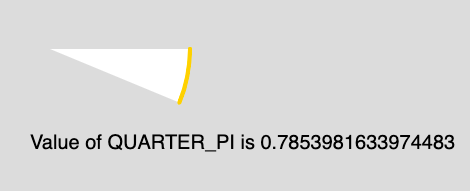Open in App
Not now

# p5.js | Constants | QUARTER_PI

• Last Updated : 10 Apr, 2019

The QUARTER_PI is a mathematical constant with the value 0.7853982. It is one quarter the ratio of the circumference of a circle to its diameter.

Syntax:

`QUARTER_PI`

Below program illustrates the QUARTER_PI constant in p5.js:

Example: This example describes the QUARTER_PI constant.

 `function` `setup() { ` ` `  `    ``// Create Canvas of size 880*300 ` `    ``createCanvas(880, 300); ` `} ` ` `  `function` `draw() { ` `     `  `    ``// Set the background Color ` `    ``background(220); ` `     `  `    ``// Set the stroke color ` `    ``stroke(255, 204, 0); ` `     `  `    ``// Set the stroke weight ` `    ``strokeWeight(4); ` `     `  `    ``// Use of constant QUARTER_PI ` `    ``arc(50, 50, 280, 280, 0, QUARTER_PI/2);  ` `     `  `    ``noStroke(); ` `     `  `    ``// Set font size ` `    ``textSize(20); ` `     `  `    ``// Display result ` `    ``text(``"Value of QUARTER_PI is "`  `            ``+ QUARTER_PI, 30, 150); ` `} `

Output:My Personal Notes arrow_drop_up
Related Articles